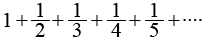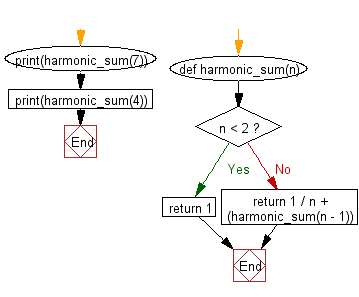﻿ Python Data Structures and Algorithms - Calculate the harmonic sum - w3resource# Python Data Structures and Algorithms - Recursion: Calculate the harmonic sum

## Python Recursion: Exercise-8 with Solution

Write a Python program to calculate the harmonic sum of n-1.
Note: The harmonic sum is the sum of reciprocals of the positive integers.

Example:Sample Solution:-

Python Code:

``````def harmonic_sum(n):
if n < 2:
return 1
else:
return 1 / n + (harmonic_sum(n - 1))

print(harmonic_sum(7))
print(harmonic_sum(4))
```
```

Sample Output:

```2.5928571428571425
2.083333333333333
```

Flowchart:## Visualize Python code execution:

The following tool visualize what the computer is doing step-by-step as it executes the said program:

Python Code Editor:

What is the difficulty level of this exercise?

Test your Programming skills with w3resource's quiz.

﻿

## Python: Tips of the Day

Decapitalizes the first letter of a string:

Example:

```def tips_decapitalize(s, upper_rest=False):
return s[:1].lower() + (s[1:].upper() if upper_rest else s[1:])
print(tips_decapitalize('PythonTips'))
print(tips_decapitalize('PythonTips', True))
```

Output:

```pythonTips
pYTHONTIPS
```